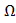# In the given circuit the internal resistance of the 18V cell is negligible.  If R1=400, R3=100and R4=500and the reading of an ideal voltmeter across R4 is 5V, then the value R2 will be:a. 300b. 230c. 450d. 550## Question ID - 50181 :- In the given circuit the internal resistance of the 18V cell is negligible.  If R1=400, R3=100and R4=500and the reading of an ideal voltmeter across R4 is 5V, then the value R2 will be:a. 300b. 230c. 450d. 5503537V4=5V

i1=V3=i1R3=1V

V3+V4=6v=V2

V1+V3+V4=18V

V1=12V

I=I2=0.2Amp

V2=6V

R2=Next Question :

A mass of 10kg is suspended vertically by a rope from the roof.  When a horizontal force is applied on the rope at some point, the deviated at an angle of 450 at the roof point.  If the suspended mass is at equilibrium, the magnitude of the force applied is (g=10ms−2)

 a. 200N b. 100N c. 140N d. 70N